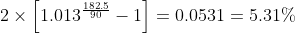Scan QR code or get instant email to install app

Question:

# Jane Acompora is calculating equivalent annualized yields based on the 1.3% holding period yield of a 90-day loan. The correct ordering of the annual money market yield (MMY), effective yield (EAY), and bond equivalent yield (BEY) is:

A MMY < BEY < EAY.
explanation

1212No calculations are really necessary here since the MMY involves no compounding and a 360-day year, the BEY requires compounding the quarterly HPR to a semiannual rate and doubling that rate, and the EAY requires compounding for the entire year based on a 365-day year. A numerical example of these calculations based on a 90-day holding period yield of 1.3% is: the money market yield is 1.3% x 360 / 90 = 5.20%, the bond equivalent yield is, which is two times the effective semiannual rate of return, and the effective annual yield is. Calculating the semiannual effective yield using 180 days instead of 182.5 does not change the order.